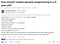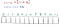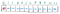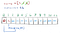# Solving Minimum Coin Change

For many people that are preparing for coding interviews, the fear of dynamic programming (DP) questions is quite common. This is in part due to the type of problems that often requires DP to solve. These questions often concern combinatorics and repetitive calculations.

Now, if someone was learning about DP, it’s likely that they would come across the minimum coin change problem (or at least a variant of it). In this post, we’ll discuss the logic involved in solving this problem.

But before we can dive into the problem, let’s talk a bit about what dynamic programming is.

# A Brief Explanation of DP

Here’s a Quora answer that I believe nails the philosophy of dynamic programming:https://qr.ae/pNouZt

Simply put, DP is a method in which we store previously calculated values so that we can easily retrieve them again without having to recalculate.

Being able to store values allows us to quickly retrieve them and use as smaller sub-solutions to solve for even larger ones.

Now, I believe what makes DP problems so frightening is not so much the method of approach, but the nature of the problem and exactly how to apply DP.

In order to better understand it, let’s look at the minimum coin change problem.

# Defining the Problem

The minimum coin change problem goes as follow:

Suppose you’re given an array of numbers that represent the values of each coin.* Then you’re given an amount and asked to find the minimum number of coins that are needed to make that amount.

* Assume the number of coins you have are infinite, so you don’t need to worry about how many coins are at your disposal.

Example:

Coins: [1, 2, 5]

Amount: 11

Answer: 3 coins (because 5 + 5 + 1 = 11)

# Approaching the Problem

From a glance, this problem seems really daunting. An initial thought might be to determine which of the combinations of coins will have the minimum number of coins.

Using this thinking, we’d determine that 11 can be made up in the following ways:

• 1 + 1 + 1 + … + 1 = 11
• 1 + 1 + 1 + 1 + 1 (9 ones) + 2 = 11
• 5 + 5 + 1= 11

Now, this approach looks rather simple. But as you can see, I got tired of writing the various combination of coins. Just imagine if we have a larger array of coins and an even larger amount. This approach would be impractical.

As a matter of fact, it’s unnecessary and tedious to record all the combination of coins. But fortunately, there’s a much simpler and arguably more elegant solution.

## DP Approach

Recall the Quora answer from earlier. Let’s start with:

1 + 1 + 1 +1 + … + 1 = 10

Now, the first time, it may take a while to add up all the 1s to get 10. But, if I was to ask you “How could you make 11?”, you would be able to tell me right away that all we have to do is: 10+ 1 = 11.

Remember, DP consists of storing previously calculated values to prevent repetitive calculations and allow for quick retrievals as well as using smaller values to solve for even larger ones.

But exactly how are we going to store our previous calculations?

Well, we could use an array. Suppose that we have this array of numbers, that will hold the minimum number of coins for each amount, starting from 0 to the amount, which in this case is 11:Each value in the array represents the minimum number of coins for each amount

Now the question we face is, what values do we initialize each index with? Well, since we’re dealing with minimums, values are often initialized to Infinity (∞). The logic is that at this moment, the minimum number of coins to make each amount is infinite:

But, there’s one important thing to note. Assuming that we’re only given positive value coins, we know that it is impossible to make an amount of 0 using any of the coins. So, we can say that there are 0 ways of making 0:There are 0 ways to make an amount of 0 with positive coin values

Okay, now let’s look at each coin separately to see how many of a specific coin can make each amount. Since our first coin is the 1-coin, we’re going to ask: “Using just 1-coins, how many coins does it take to make a value of amount: 1? 2? … 11?”

First, let’s ask: “From 0, how many 1-coins do I need to make 1?”

Well, that’s simply 1.

0 + 1 = 1

Now, we ask: “Okay now, which is smaller? 1 or ∞ ?”

It’s 1.

Let’s visualize it:The 1 in the min() represents an additional coin, NOT a 1-coin

What we’re basically doing is we’re looking at how many coins it takes to make amount 0 and then using that amount to make 1. Another way of thinking about it is if we imagined having a pile of coins that make up 0 and then adding another coin to make 1.

Then, we determine if the new pile uses up less coins than the current current. Since 1 < ∞, we replace ∞ with 1.

We repeat this process with 2, 3, … , 11:

Here, we’re saying that we already know that it takes one 1-coin to make the amount 1. In order to make amount 2, we just put a 1-coin on top of the pile that makes up amount 1 and determine if that new pile is less than ∞. If so, we replace ∞ with the new minimum number of coins.

Okay, before I go to the next step, you’re probably asking: “What about the other coins, 2 and 5?” We’re getting to them next. What I wanted to emphasize is the idea that we’re solving this problem one coin at a time. Using just one coin, we ask ourselves: “Using just this coin, what’s the minimum number of coins that we can use to get this specific amount?

Now, let’s look at what happens when use both 1 and 2. We’ve already seen the ways in which using 1-coins will get us a certain amount. But now, we’re going to look at whether we can replace some of those 1-coins with 2-coins.

It’s clear that we can’t make the amounts 0 and 1 using a 2-coin. So those values stay the same. But what about the amount 2?

Since we can now use a 2-coin, can’t we just use that coin instead of using two 1-coins? As a matter of fact, isn’t this just the same as having an amount of 0 and then adding a 2-coin to make the amount 2?:

0 + 2 = 2 (using one 2-coin)

Now, that we can use 2-coins, we can ask how we can make 3 using both coins 1 and 2. Well, we already know how to make 3 using just 1-coins. But, what about 1-coins and 2-coins? Well, we can:

1 + 2 = 3

Or simply put, from the amount 1, we can put a 2-coin on top of it to make 3:

Now, this is where it gets interesting and I think you might start to see the pattern emerge. In order to create the amount 4 using 1-coins and 2-coins, we can see:

• 1 + 1 + 1 + 1 = 4 (only 1s)
• 1 + 1 + 2 = 4 (both 1 and 2)
• 2 + 2 = 4 (all 2s)

As we can see, that using only two 2-coins will give us the minimum number of coins it takes to make the amount 4. Or another way to put it, from the amount 2, we can add a 2-coin to make 4:

From amount 6, we can add a 2-coin. That will give us an amount of 8. Since the minimum number of coins needed to make 6 is 3 (2 + 2 + 2), the new minimum number of ways to make 8 is by putting a 2-coin on top of the amount 6, thus making it 4 coins.

Now that we’ve finished looking at the minimum number of coins needed to make each amount using 1-coins and 2-coins, let’s look at 5-coins:

It’s becoming clear that the first argument in the min() is array[current_amount — current_coin] + 1, while the second argument is just the array[current_amount].

And at last, we’ve exhausted all our coin options and see that at amount 11, there is a minimum value of 3 coins (5 + 5 + 1) that are required to make 11.

Now, let’s look at the code.

# Code

The code is written as follow using JavaScript:

# Analysis

From the code, we can see that we’re using two for-loops. Since we’re iterating over the entire minCoins (which has a length equal to amount) array every time we iterate over an element in the coins array, we say that the runtime of this algorithm is O(|coins|•|amount|) where |coins| is the length of the coins array and |amount| is the length of the minCoins array.

And since we’re using an array to keep track of our minimum coins for each amount, minCoins, the space complexity is O(|amount|).

# Summary

• Dynamic Programming (DP) is simply the method of storing previously calculated values so that we don’t have to recalculate them, which saves us time and allows us to use smaller sub-solutions to solve larger ones.
• Look at one coin at a time and find out what is the minimum number of coins that are needed to make each amount from 0 to amount.
• Runtime: O(|coins|•|amount|), where |coins| represent the length of the coins array and |amount| represents the length of minCoins array.
• Space Complexity: O(|amount|) because we used an array to keep track of the minimum number of coins for each amount.

## More from Try Khov

Software Engineer